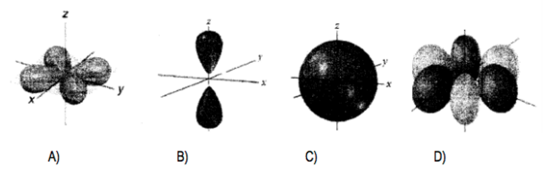# Problem: Which of the following illustrates an orbital with quantum numbers n = 5, l = 1?

###### FREE Expert Solution
100% (429 ratings)
###### Problem Details

Which of the following illustrates an orbital with quantum numbers n = 5, l = 1?What scientific concept do you need to know in order to solve this problem?

Our tutors have indicated that to solve this problem you will need to apply the Quantum Numbers: Angular Momentum Quantum Number concept. You can view video lessons to learn Quantum Numbers: Angular Momentum Quantum Number. Or if you need more Quantum Numbers: Angular Momentum Quantum Number practice, you can also practice Quantum Numbers: Angular Momentum Quantum Number practice problems.

What is the difficulty of this problem?

Our tutors rated the difficulty ofWhich of the following illustrates an orbital with quantum n...as low difficulty.

How long does this problem take to solve?

Our expert Chemistry tutor, Jules took 1 minute and 55 seconds to solve this problem. You can follow their steps in the video explanation above.

What professor is this problem relevant for?

Based on our data, we think this problem is relevant for Professor Sotero's class at UCF.